## Recommended Posts

I don’t understand interference.  Do you?

First, some background.  It was found, empirically, that when we send a certain kind of stuff (“particles,” such as photons or electrons) through a very narrow slit in a plane, and we detect them on a screen that is parallel to and far away from the plane, we find that individual particles are detected, and if we detect enough of them, their distribution forms what is called the Fraunhofer diffraction approximation:

In the above example, we assume that the source of the particles is either narrow enough or sufficiently far away that the transverse momentum component of the incoming particles is sufficiently close to zero so that the diffraction pattern is primarily the result of a change in the transverse momentum caused by passing through the slit.

It was also found, empirically, that if we send the same kind of particles through two narrow slits (say, a left slit and a right slit) in a plane, separated by small distance, we find that the particles detected on a far-away screen that is parallel to the plane form what is called an interference pattern:

Notice that the interference pattern seems like it could fit inside the diffraction pattern shown earlier; we call this the diffraction envelope.  In the above example, the distance between the slits was about four times the slit width, and the greater this ratio, the narrower the distance between peaks inside the diffraction envelope.

Let me reiterate something: individual particles are detected at the screen.  And if we slow down the experiment adequately, we will see individual “blips” on the screen.  None of these blips is, itself, a distribution pattern; rather, the distribution pattern (diffraction in the case of one slit or interference in the case of two) becomes apparent only after measuring lots of lots of blips.

Immediately a problem arises in the case of interference.  Since individual particles are both emitted by the source and detected at the screen, it certainly seems plausible that individual particles pass through the slits.  However, if a particle passes through, say, the left slit, then it should produce a single-slit diffraction pattern, unless the particle somehow “knows” about the existence of the right slit.  Because an interference pattern is actually created, then either:
a) The particle, as it passes through the left slit, must “instantly” know about the existence and size of the right slit, which is located some distance away; or
b) It is not the case that the particle passes through the left slit (or the right slit, by similar reasoning).

The problem with a) is nonlocality.  Special Relativity asserts that information cannot travel faster than the speed of light, which implies that instantaneous transfer of information is impossible.  Historically, Special Relativity was proposed by Einstein in 1905, about two decades before the formal creation of quantum wave mechanics.  So option a) was summarily dismissed on the grounds that the path of a particle (in this case the transverse momentum component of a particle passing through the left slit) could not possibly be affected by nonlocal information about the right slit located some arbitrary distance away.

Consequently, we have been stuck, for nearly a century, with option b).  How is it the case that there is no particle that passes the left slit or the right slit, even though a particle was emitted by the source and detected at the screen?  Herein lie both the mathematical beauty and the philosophical wackiness of quantum mechanics.

Essentially, quantum wave mechanics begins by assuming that the likelihood of finding a particle at a location in space is related to the magnitude of a wave at that point.  Because one-dimensional waves are sinusoidal, standing waves have the form eikx.  In the case of single-slit diffraction, a wave originating at the slit will spread out radially so that the wave, as measured along the transverse direction, will vary sinusoidally but will also decrease linearly as the radial distance r from the slit.  When the distance from the slit is determined almost entirely by the distance between the slit and the screen (i.e., the diffraction angle is small, such as 1°), then the wave in the x direction varies like sin(αx)/αx (also known as sinc(αx)).  Then, the likelihood of actually detecting a particle on the screen between two locations is just found by integrating the probability distribution ρ(x) = Ψ*(x) Ψ(x), which looks like the experimentally observed Fraunhofer diffraction, shown previously.

Now let’s apply this mathematical formalism to the double-slit problem.  We now assume that at the location of the plane of the slits we can represent the (particle?) system as a wave Ψ consisting of the superposition of a left-slit wave ΨL and a right-slit wave ΨR so that Ψ(x) = ΨL(x) + ΨR(x).  The beauty of this equation is that if we now plot the probability distribution ρ(x) = Ψ*(x) Ψ(x), we get what looks like the experimentally observed interference distribution, shown previously.  In other words, if we assume that the system at the location of the slits is not a particle, but a wave that later determines probabilities of detection, then we successfully predict the empirically observed probability distributions.

The reason this works, mathematically, is because quantum wave mechanics allows “negative” probabilities.  Look back at the interference distribution and choose some place on it where the probability is zero.  If only one slit had been open, the probability of detecting a particle at this point would have been nonzero.  So how is it that by adding another slit – by adding another possible path through which a particle could reach that point – that we decrease its likelihood to reach that point?  The answer, mathematically, is that by adding waves prior to taking their magnitude, terms that are out of phase can cancel each other, resulting in a sort of negative probability.

However, something doesn’t make sense.  Remember that the wave ΨL(x) is associated with a particle that travels through the left slit and wave ΨR(x) is associated with a particle that travels through the right slit.  But what can this possibly mean if we have already assumed that it is not the case that the particle passes through the left slit or the right slit?

This is the very heart of the so-called “measurement problem.”  By localizing the particle (or whatever the hell it is) within the two slits, we assume that it is in superposition Ψ(x) = ΨL(x) + ΨR(x).  But if we subsequently measure the particle as having come from one of the slits (called a “which-way” measurement), then we were wrong about its earlier state.  And if we were right about its earlier state, then it will forever remain in a superposition, unless we allow for nonlinear, irreversible "collapse," whatever the hell that is.

So there is something very weird, and possibly wrong, with option b).  So maybe option a) is right and we just have to accept nonlocality.  After all, quantum entanglement also seems to require nonlocality, so maybe that’s just a fact about the quantum world.  Physicist Yakir Aharonov has written a lot on the topic of nonlocality in quantum measurement, such as this.

By the way, treating a system with two slits as the superposition of two waves still does not solve the nonlocality problem.  After all, consider a single slit of width Δx.  This produces a single-slit Fraunhofer diffraction distribution and certainly no one would object to the assertion that every particle detected on the screen actually passed through the slit.  (Right??)  Of course, if Δx is zero, then there’s no problem with Special Relativity.  However, no slit has zero width, so let’s divide the slit into a left half and a right half.  Now, we can associate a wave with each half and treat each half as producing its own Fraunhofer diffraction envelope having double the width.  The interference between these two waves then produces an interference pattern that, incredibly enough, is identical to the single-slit Fraunhofer diffraction distribution of the entire slit.  In other words, a single-slit diffraction is double-slit interference for side-by-side slits.  So we are again left with options a) and b), even for a single slit.

It is important to remember that the representation of the system as a complex superposition of mutually exclusive possibilities was (and remains) an assumption.  Of course, it is an assumption whose numerical predictions have been empirically tested and confirmed to staggering precision.  However, if there is an understanding of the quantum world that yields the same or better predictions while avoiding sloppy philosophical paradoxes, then might that be preferred?

I’m proposing a different approach.  I do not think it’s original, but frankly after lots of research, I can’t find this approach.

First, consider a localization experiment of a particle, and let’s assume that the particle actually is located somewhere.  In the case of a single slit, let’s assume that at some time the particle is, in fact, located somewhere in the slit with constant probability; in the case of the double slit, it is located in either slit with equal probability; and so forth.

Next, take the Fourier transform of the entire location distribution, then take its magnitude.  For some reason, for a square function (corresponding to a single slit), this yields exactly the Fraunhofer diffraction distribution in momentum space.  We can then empirically find the relationship between the momentum space and position space by noting that the spread of the distribution in momentum space is inversely proportional to the spread in position space, and their product is on the order of Planck’s constant.

By the way, I don’t yet understand why the magnitude of the Fourier transform of a square function yields the sinc2(αx) distribution so typical of Fraunhofer diffraction, although I suspect it generalizes by starting from a “perfect” localization down to the Planck length (and the resulting complete lack of knowledge one could have about momentum at this scale).  In any event, not only do I find this fact amazing, but I frankly wasn’t convinced that p=ℏk was the same momentum as p=mv of a massive object until I noticed that distributions of particles passing through a slit correspond to the magnitude of the Fourier transform of that slit!

Finally, assume each particle passing through the localized region can take on transverse momenta according to this distribution, and then integrate this value over the entire localized region.  This, I believe, may yield the actual distribution of detected particles.

To test my results, I used Mathematica to simulate the situations numerically.  In each case I divided the localization region into lots of smaller regions.  In one case (called “Adding Fields”), I added the fields of all the regions first before calculating intensities/probabilities; in the other case (called “Adding Probabilities”), I calculated intensities/probabilities first and then added the contributions by each region.  I made a few assumptions:
·         The incoming particles had momentum such that the central diffraction envelope spreads at an angle of 1°.
·         The incoming particles were assumed to come from a point source with effectively no spread in momentum (which, I think, is another way of saying they are assumed to be monochromatic and spatially coherent).

For diffraction, I divided the single slit into n regions.  In the Adding Fields simulation, I calculated the Fourier transform of each region to find their fields, added the fields of each region, and then plotted the magnitude of this sum for various parameters.  In the Adding Probabilities simulation, I calculated the Fourier transform of the entire slit, assumed that each region produces an intensity based on the fields in this total Fourier transform, and then plotted the sum of these intensities for various parameters.  Here is a typical example, in which the slit is divided into 20 regions and the screen is a distance of 50 times the slit width:

A distance of 50 times the slit width is very much in the near field, where we would expect the distribution to be relatively flat (corresponding to the width of the slit), with edge effects that reflect the 1° spread.  Only the Adding Probabilities distribution satisfies these expectations.  The situation is worse when the slit is divided into 100 regions:

In the far field, such as where the screen is 10,000 times the slit width, both simulations converge to the expected Fraunhofer diffraction distribution:

To simulate double-slit interference in the Adding Fields simulation, I simply added another slit of equal width, some distance away, and broken into n regions, and continued the analysis by first adding the fields of each region and then finding the magnitude of their sum.  In the Adding Probabilities simulation, I calculated the Fourier transform of both slits together (i.e., the entire localization space), assumed that each region produces an intensity based on the fields in this total Fourier transform, and then plotted the sum of these intensities for various parameters.  Here is a typical example, in which the slits are each divided into 100 regions, the slit separation is 10 times the slit width, and the screen is located a distance of 10 times the slit width.  The plots are also shown zoomed in to the left peak:

Either of these distributions might fit experimental data, however the Adding Probabilities distributions are more plausible.  In the far field, starting at around a million times the slit width, both simulations converge to the expected interference pattern:

For the life of me, I CANNOT FIND THE ANSWER.  I have read dozens of papers and scoured the internet, and basically every source says that you add the fields first and then find the probabilities, instead of just doing a Fourier transform on the entire localization space and assuming that each localized particle assumes the resulting momentum distribution.  That, or I'm just not understanding what I'm reading.  This method is also pretty simple, so I seriously doubt I’ve discovered something new... which means I must have made a mistake somewhere.  There are certainly references (such as this) that say that you add probabilities when the source particles are incoherent, but my analysis seems to apply to any source, including a laser.

##### Share on other sites

Fields (such as the electric field you find in EM radiation) are vectors and you add them when two are superimposed. If they have opposite signs, they will cancel (partly or fully, depending on the amplitudes) when added.

Intensities (and probabilities) are found by squaring the field.

17 hours ago, aknight said:
Immediately a problem arises in the case of interference.  Since individual particles are both emitted by the source and detected at the screen, it certainly seems plausible that individual particles pass through the slits.  However, if a particle passes through, say, the left slit, then it should produce a single-slit diffraction pattern, unless the particle somehow “knows” about the existence of the right slit.  Because an interference pattern is actually created, then either:

a) The particle, as it passes through the left slit, must “instantly” know about the existence and size of the right slit, which is located some distance away; or
b) It is not the case that the particle passes through the left slit (or the right slit, by similar reasoning).

The individual electron or photon is not a tiny ball. Both will have a wave nature, and the wave passes through both slits. The particle can interfere with itself. The interference and diffraction will dictate where the particle can, and can't, be detected.

17 hours ago, aknight said:

Now let’s apply this mathematical formalism to the double-slit problem.  We now assume that at the location of the plane of the slits we can represent the (particle?) system as a wave Ψ consisting of the superposition of a left-slit wave ΨL and a right-slit wave ΨR so that Ψ(x) = ΨL(x) + ΨR(x).  The beauty of this equation is that if we now plot the probability distribution ρ(x) = Ψ*(x) Ψ(x), we get what looks like the experimentally observed interference distribution, shown previously.  In other words, if we assume that the system at the location of the slits is not a particle, but a wave that later determines probabilities of detection, then we successfully predict the empirically observed probability distributions.

You can't break up the wave function in that way.

##### Share on other sites

There are several inaccuracies in the first post. Among others:

eikx is for a propagating wave, not a standing one.

The amplitude psi does not decay with distance r as 1/r after exiting a slit. This would be for a point or spherical source. In the zone useful for interference, the frontwave area increases like a cylindrical area does, and so does psi2 decrease, because it varies like a power density, since a photons is thought like an energy quantum.

Beware with the idea of local detection ! This is badly difficult to understand in QM, most book convey a wrong interpretation. The simultaneous histories of a photon don't vanish upon detection. The double slit experiment is misleading in this aspect. You could check different experiments, for instance interferences of atoms that both have or have not absorbed a first photon and then can absorb a second one: the observed atom interferences tell that after the first light ray, the atom is in both states, having absorbed a photon and not. Consequently, said photon too is on both states, having been absorbed and not.

More generally, starting QM with the double slit is a very bad idea. Starting with the wave function of pentacene observed by atomic force microscopy would be better. It would show that the wave function is observed, and that the same pair of electrons is observed over the whole molecule size without any destruction.

Causality and propagation delay: no huge difficulty. At  least two answers cope with it:
- External objects, including humans, have no influence. Then the simultaneity does not transport information, because no information can be encoded. Faster than light is then possible.
- There has been no collapse at all of the wavefunction. This is the preferred explanation now, especially in light of newer experiments like the eraser. All possible events did happen, so no information transfer is necessary. What looks like a collapse is only that the observer, if he is in the state having made the observation A, does not feel its states having made the observations B because there are so many and uncorrelated that they sum up to zero.

Negative probabilities aren't needed. As Swansont said, |psi|2 is computed after summing all necessary psi. This is what lets waves interfere and tells us that photons or electrons are waves.

Beware with  the destruction of interferences by knowing "which slit". Meanwhile, "weak measurements" have been experimented, and "knowing" isn't binary.

That two apertures half as wide work as one of full width is Huygen's principle. And, yes, diffraction exists with single apertures too, for instance optical lenses. The computation method, summing over all possible positions, is the standard one.

sinc just results from summing a uniform illumination over a window width. You can just forget the dimension along the slit for that and compute 1D. Write the phase shift along the width of the slit due to the considered propagation direction, you get the sinc.

"Zero momentum information" from a point source is wrong. If the particle has a spin, for instance the photon has, then the directions perpendicular to the (emitted or selectively detected) straight spin are more strongly illuminated (stronger psi2 blah blah blah) while the direction aligned with the spin aren't at all, and in between the pattern is a cosine.

I'll stop here for lack of time, apologies, even before having gotten a general sense of the thesis. Strong encouragements to go on thinking by yourself, because most explanations about QM are badly wrong. Most books just reproduce the misunderstandings of the very early times of QM, before decisive experiments were done. Only personal thinking can debug that, and is consumes horribly much time, alas. My suggestion is to consider soon other experiments than the double slit, among them, images of the pentacene by atomic force microscope (search words).

##### Share on other sites
34 minutes ago, Enthalpy said:

There are several inaccuracies in the first post. Among others:

eikx is for a propagating wave, not a standing one.

The amplitude psi does not decay with distance r as 1/r after exiting a slit. This would be for a point or spherical source. In the zone useful for interference, the frontwave area increases like a cylindrical area does, and so does psi2 decrease, because it varies like a power density, since a photons is thought like an energy quantum.

Beware with the idea of local detection ! This is badly difficult to understand in QM, most book convey a wrong interpretation. The simultaneous histories of a photon don't vanish upon detection. The double slit experiment is misleading in this aspect. You could check different experiments, for instance interferences of atoms that both have or have not absorbed a first photon and then can absorb a second one: the observed atom interferences tell that after the first light ray, the atom is in both states, having absorbed a photon and not. Consequently, said photon too is on both states, having been absorbed and not.

More generally, starting QM with the double slit is a very bad idea. Starting with the wave function of pentacene observed by atomic force microscopy would be better. It would show that the wave function is observed, and that the same pair of electrons is observed over the whole molecule size without any destruction.

Causality and propagation delay: no huge difficulty. At  least two answers cope with it:
- External objects, including humans, have no influence. Then the simultaneity does not transport information, because no information can be encoded. Faster than light is then possible.
- There has been no collapse at all of the wavefunction. This is the preferred explanation now, especially in light of newer experiments like the eraser. All possible events did happen, so no information transfer is necessary. What looks like a collapse is only that the observer, if he is in the state having made the observation A, does not feel its states having made the observations B because there are so many and uncorrelated that they sum up to zero.

Negative probabilities aren't needed. As Swansont said, |psi|2 is computed after summing all necessary psi. This is what lets waves interfere and tells us that photons or electrons are waves.

Beware with  the destruction of interferences by knowing "which slit". Meanwhile, "weak measurements" have been experimented, and "knowing" isn't binary.

That two apertures half as wide work as one of full width is Huygen's principle. And, yes, diffraction exists with single apertures too, for instance optical lenses. The computation method, summing over all possible positions, is the standard one.

sinc just results from summing a uniform illumination over a window width. You can just forget the dimension along the slit for that and compute 1D. Write the phase shift along the width of the slit due to the considered propagation direction, you get the sinc.

"Zero momentum information" from a point source is wrong. If the particle has a spin, for instance the photon has, then the directions perpendicular to the (emitted or selectively detected) straight spin are more strongly illuminated (stronger psi2 blah blah blah) while the direction aligned with the spin aren't at all, and in between the pattern is a cosine.

I'll stop here for lack of time, apologies, even before having gotten a general sense of the thesis. Strong encouragements to go on thinking by yourself, because most explanations about QM are badly wrong. Most books just reproduce the misunderstandings of the very early times of QM, before decisive experiments were done. Only personal thinking can debug that, and is consumes horribly much time, alas. My suggestion is to consider soon other experiments than the double slit, among them, images of the pentacene by atomic force microscope (search words).

Thanks for the comments.  I read them and will look closer.  And I appreciate the details, but yes, you've missed the general/overall point.  I summarized it here and I would be curious about your thoughts:

Consider a double-slit experiment.  The following normalized probability distribution shows the localization of a particle in a region defined by two slits.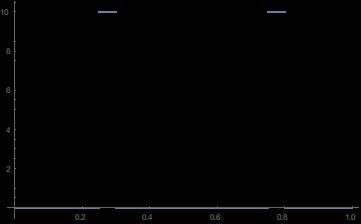Fig. 1 Probability distribution in plane of double-slit.

The traditional way of calculating distributions on a detector screen would be to assume that each point in the slits is a point source of waves (or complex fields).  These waves add together (or superpose), and when particles are finally detected at a screen, we find the probabilities by finding the magnitude of the superposed waves.  In the far field, we get exactly what we expect: an interference pattern.  However, in the near field, we get something weird.  This is what it looks in front of one slit in the near field: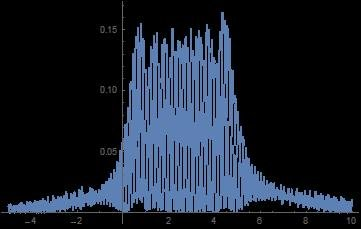Fig. 2.  Distribution in near field of slit according to traditional method.

The method I am suggesting is to assume that each point in the slits is a point source of intensity (not waves), where the intensity is found by taking the magnitude of the Fourier transform of the total region of localization.  The magnitude of the Fourier transform of the probability distribution in Fig. 1 is: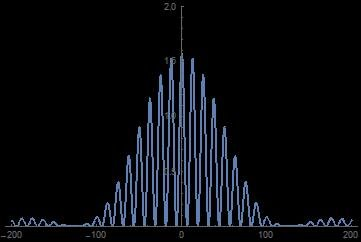Fig. 3.  Magnitude of Fourier Transform of distribution in Fig. 1.

In the far field, we get exactly the same interference pattern as by the traditional method (which is what we would expect), but in the near field, this is what it looks like in front of one slit.  (Ignore the units as I forgot to normalize...)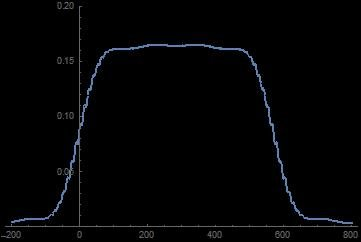Fig. 4.  Distribution in near field of slit according to proposed method.

The situation is worse for the traditional method for single-slit diffraction because not only is it messy (like Fig. 2) but its spread is much too large.  (The numerical simulations assumed a dispersion of 1°.)  Fig. 5 shows the near field (where the distance to the screen is only 50 times the slit width) of the traditional method and Fig. 6 shows if we treat each point in the slit as a point source of intensity (instead of waves).  My question is this: what distribution would we expect if we placed a detector screen right up near a slit?  Seems obviously Fig. 6, right?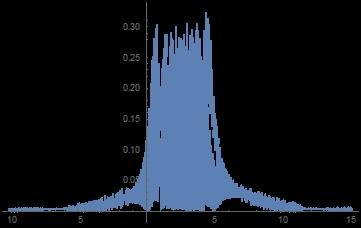Fig. 5.  Distribution in near field of one slit according to traditional method.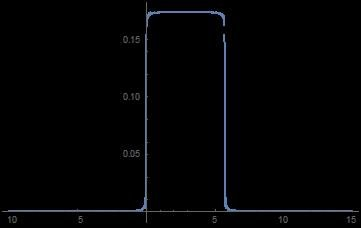Fig. 6  Distribution in near field of one slit according to proposed method.

## Create an account

Register a new account